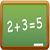PART66 Licensing Info
Licence Categories
Modularization
Number of questions
Experience requirements
Licence extension

Remember me

RegistrationModule 1. Mathematics
What is the characteristic of 5.74?
•  -1
•  0
•  1

•  whats 5.74 ?

dimky
Posts: 509
18.01.2014 / 15:49
5.74 is a number.
satishpandey
Posts: 6
18.01.2014 / 16:59
thanks
piesoup
Posts: 3
08.01.2015 / 21:27
If you find the Log of 5.74, it will be 0.--- The '0' is the characteristic.
Paulo Santos
Posts: 1
27.01.2015 / 16:15
thanks
cefoim
Posts: 1
12.01.2017 / 06:20
Don't understand the question
JD2016
Posts: 54
12.01.2017 / 22:47
Log is short for Logarithm. When someone asks what is "Log 5.74", they mean "what is the Logarithm of 5.74". They want to know what power you have to raise the number 10 to, to get 5.74.
10 to the power of 1 is 10 which is too high. 10 to the power of zero is 1 (anything to the power of zero is One!). Therefore the power must be between 0 and 1. The actual power required is 0.7589.
The characteristic is always 1 less than the number of digits to the left of (or before) the decimal point. So with 5.74, there is 1 digit on the left of the decimal point (i.e. the number 5) and therefore the characteristic is (1-1 =0).
The characteristic of 120 is 2 - the log of 120 is going to be somewhere between 2 and 3 because 10 squared is 100 and 10 cubed is 1000.
Brian Dyas
Posts: 2
29.09.2021 / 09:45
Thank you JD